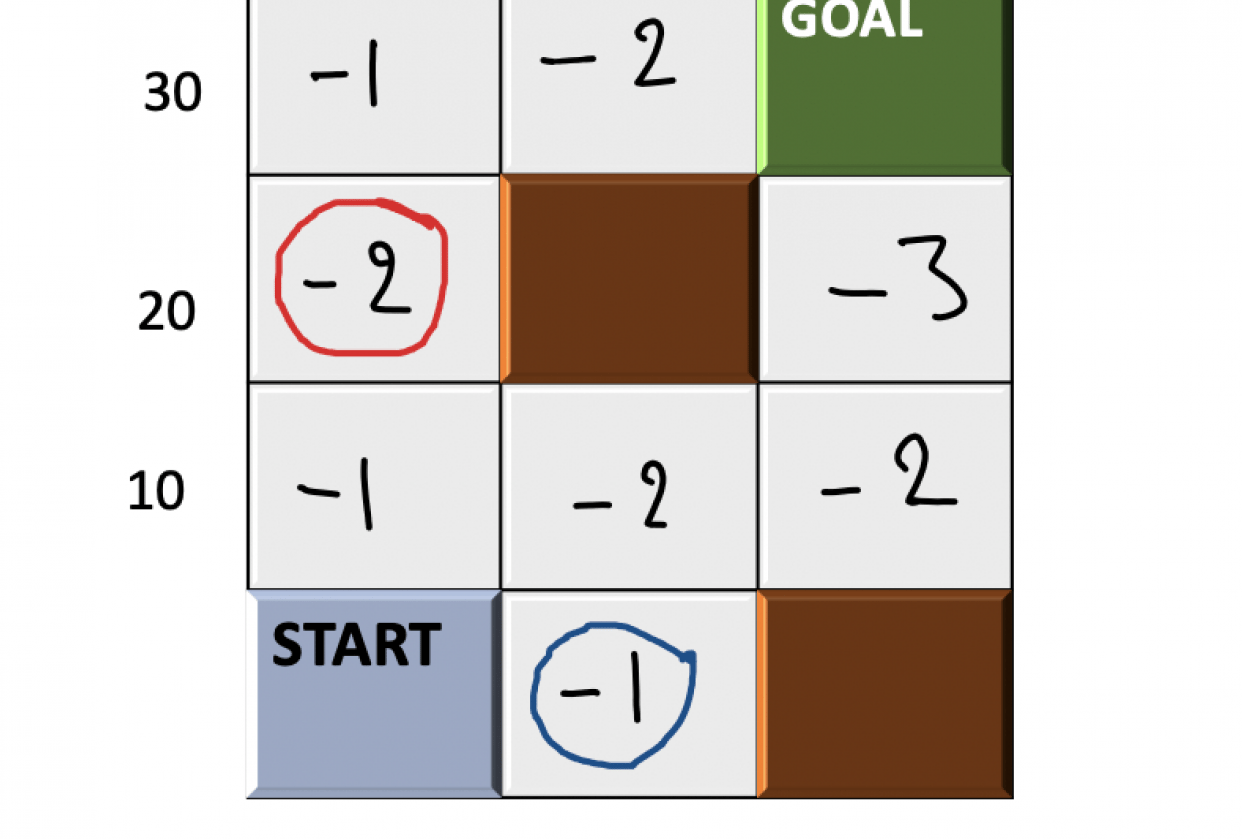## Reinforcement Learning - Evaluate States based on Value FunctionIn the given image of Grid with different Reward values, (0,0) is the Starting state and (3,2) is the Goal. The values inside the grid cells(States) represent the immediate Rewards corresponding to those states. We have studied that Value function is used to compare the goodness/badness of a state. Your task is to:
(a.) Calculate the Value function of state (2,0) circled in Red
(b.) Calculate the Value function of state (0,1) circled in Blue
(c.) Out of (2,0) and (0,1) which is a better state and why?
Here I am not providing any Policy, so use the best Policy for your calculations.